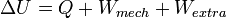# Thermodynamics: cylindrical figures contains a gas

## Homework Statement

A cylindrical figures contains a gas and we have a piston that is capable of changing the gases volume.
Initial state: V0=1l P0=10^5p T=300°K

We pressurize on the piston that has a mass m=300g and a surface S=20cm^2.
The new temperature T1=540°K

## Homework Equations

Calculate the change in internal energy.

## The Attempt at a Solution

Well I know that the change in internal energy is: ΔU=W+Q or ΔU=-P1(V1-V0)

and that p=F/S. but since my teacher didn't explain the lesson well enough I have no clue on how to answer it. Any help would be very well appreciated.

Last edited by a moderator:

i believe i would have to use this one right?I would get: ΔU=p1(V0-V2)+Q right?

Simon Bridge
Homework Helper
OK, I'll let you off the hook:
Internal energy for an ideal gas depends only on it's temperature.
$$\Delta U = \frac{3}{2}nR\Delta T$$

You know the change in temperature - it's given to you.
You need to find nR.

OK, I'll let you off the hook:
Internal energy for an ideal gas depends only on it's temperature.
$$\Delta U = \frac{3}{2}nR\Delta T$$

You know the change in temperature - it's given to you.
You need to find nR.

alright i'll give it a try right now.

I got n=.0401 by using the equation of ideal gases and i got a change of internal energy as 55J correct?

Last edited:
I got n=.0401 by using the equation of ideal gases and i got a change of internal energy as 24.5J.

Is this correct?

Simon Bridge